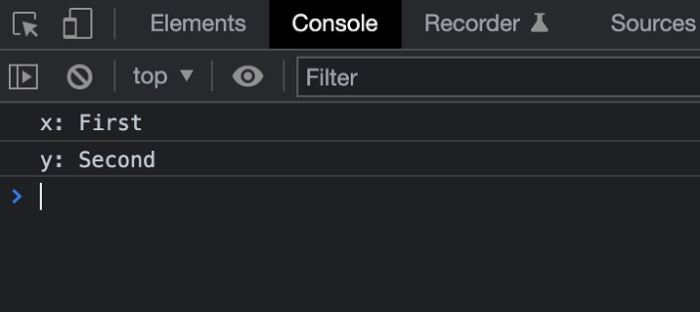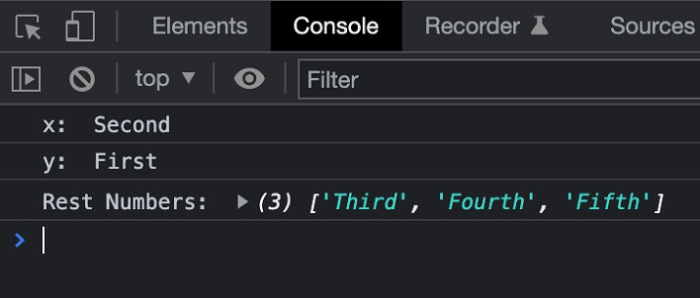# How to swap variables using Destructuring Assignment in JavaScript?

The Destructuring assignment is a feature that was introduced in the ECMAScript 2015. This feature lets the user extract the contents of the array, and properties of an object into distinct variables without actually writing the repetitive code

This assignment lets the expression unpack values from arrays, and properties into distinct variables.

## Example 1

In the below example, we use the destructuring assignment to assign variables. In the example, we have two variables defined as first and second. In the method, we are destructing the variables to assign the variables of the array to x and y respectively.

# index.html

<!DOCTYPE html>
<html>
<title>Checking If a Number is Even</title>
<body>
<h1 style="color: green;">
Welcome To Tutorials Point
</h1>
<script>
let x;
let y;
let arr = ["First", "Second"];
[x, y] = arr;
console.log("x:", x);
console.log("y:", y);
</script>
</body>
</html>

## Output## Example 2

In the below example, we are going to assign the value first as done in the above example. Once the values are assigned we will swap these values and then assign them to x and y.

# index.html

<!DOCTYPE html>
<html>
<title>Checking If a Number is Even</title>
<body>
<h1 style="color: green;">
Welcome To Tutorials Point
</h1>
<script>
let x;
let y;
let arr = ["First", "Second", "Third", "Fourth", "Fifth"];
[x, y, ...rest] = arr;
[x, y] = [y,x];
console.log("x: ", x);
console.log("y: ", y);
console.log("Rest Numbers: ", rest);
</script>
</body>
</html>

## Output